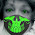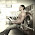## Friday, May 20, 2016

### Cover Reveal: Island Counselor by Sue Brown=•=•=•=•=•=•=•=•=•=•=•=•=•=•=•=•=•=•=•=•=•=•=•=•=•=•=•=•=

Sue Brown reveals the sexy cover art of her next book titled ISLAND COUNSELOR coming out from Dreamspinner Press.

It releases on June 20, 2016.

=•=•=•=•=•=•=•=•=•=•=•=•=•=•=•=•=•=•=•=•=•=•=

BLURB

Counselor Logan Wilde has a successful therapy practice in London, but when a traumatic incident there leaves him suffering from PTSD, he takes refuge in his holiday cottage on the Isle of Wight, unable to face going back to work. Not that he’s allowed to relax. Logan’s time is taken up with helping Liam Owens, plus there’s Nick Brent, whom Logan discovers collapsed on the beach. Nick and Logan spend their time bickering with each other, but that doesn’t alter the attraction they both feel.

Logan is forced to make some hard decisions about his future, which entails facing up to recent events, only he’s not alone—Nick is with him. Unfortunately, someone else makes a decision, too, and now trouble is on its way to the Isle of Wight.

=•=•=•=•=•=•=•=•=•=•=•=•=•=•=•=•=•=•=•=•=•=•=•=•=•=•=•=•=

PRESENTING

Cover Art by L.C. Chase=•=•=•=•=•=•=•=•=•=•=•=•=•=•=•=•=•=•=•=•=•=•=

EXCLUSIVE TEASER
Logan took a moment to admire Nick’s wiry, muscled figure before jogging up to him. “I thought we were meeting at nine?”

“I’m used to being up early.”

“You miss being out at sea,” Logan said.

“Every ship and boat is like a stab to my heart. Every wave calls me out there.” Nick sighed, shifting restlessly. “Will I ever get back, doc?”

Check out other exclusive teasers on -

=•=•=•=•=•=•=•=•=•=•=•=•=•=•=•=•=•=•=•=•=•=•=

ABOUT THE AUTHOR

Sue Brown is owned by her dog and two children. When she isn't following their orders, she can be found plotting at her laptop. In fact she hides so she can plot and has gotten expert at ignoring the orders.

Sue discovered M/M erotica at the time she woke up to find two men kissing on her favorite television series. The series was boring; the kissing was not. She may be late to the party, but she's made up for it since, writing fan fiction until she was brave enough to venture out into the world of original fiction.

:: Website :: Blog :: Facebook :: Twitter :: Google+ ::

=•=•=•=•=•=•=•=•=•=•=•=•=•=•=•=•=•=•=•=•=•=•=

RAFFLECOPTER GIVEAWAY

Winner’s Prize: \$10 Amazon/ARe Gift Card

#### 2 comments:

1.Very appealing cover, Sue. Wish I was at that beach just about now ;)The story seems quite intriguing as well.
Congratulations on your brand new baby and much, much success to you!
taina1959 @ yahoo.com
GiveARose (former GiveARush)

2.I do love me some Sue Brown! The cover is simply amazing. Congratulations on the release and looking forward to reading it!

juliesmall2016(at)gmail(dot)com